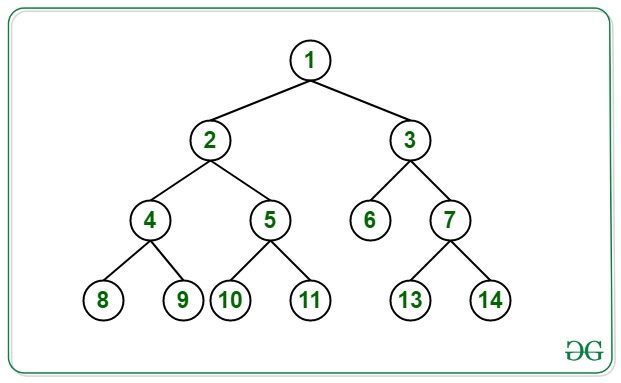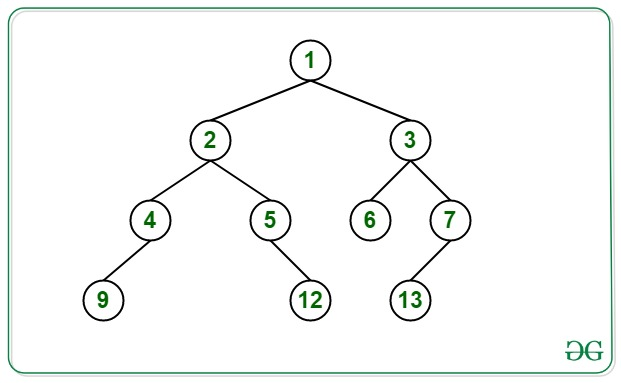# Print the nodes corresponding to the level value for each level of a Binary Tree

• Difficulty Level : Hard
• Last Updated : 22 Jun, 2022

Given a Binary Tree, the task for each level L is to print the Lth node of the tree. If the Lth node is not present for any level, print -1.

Note: Consider the root node to be at the level 1 of the binary tree.

Examples:

Input: Below is the given Tree:Output:
Level 1: 1
Level 2: 3
Level 3: 6
Level 4: 11
Explanation:
For the first level, the 1st node is 1.
For the second level, the 2nd node is 3.
For the third level, the 3rd node is 6.
For the fourth level, the 4th node is 11.

Input: Below is the given Tree:Output:
Level 1: 1
Level 2: 3
Level 3: 6
Level 4: -1
Explanation:
For the first level, the 1st node is 1.
For the second level, the 2nd node is 3.
For the third level, the 3rd node is 6.
For the fourth level, the 4th node is not available. Hence, print -1.

Approach: To solve this problem the idea is to use Multimap. Follow the steps below to solve the problem:

1. Traverse the given tree and store the level of each node and the node’s value in the Multimap.
2. The levels of the nodes are considered as the key of the multimap. Keep track of the maximum level of the Binary Tree(say L).
3. Now, iterate the Multimap over the range [1, L] and perform the following operations:
• For each level L, traverse till the Lth node of that level check if it exists or not. If found to be existing, print the value of that node.
• Otherwise, print “-1” and proceed to the next level.

Below is the implementation of the above approach:

## C++

 `// C++ program for the above approach``#include ``using` `namespace` `std;` `// Stores the level of the node and``// its value at the max level of BT``multimap<``int``, ``int``> m;` `// Stores the maximum level``int` `maxlevel = 0;` `// Structure of Binary Tree``struct` `node {` `    ``int` `data;``    ``struct` `node* left;``    ``struct` `node* right;``};` `// Function to insert the node in``// the Binary Tree``struct` `node* newnode(``int` `d)``{``    ``struct` `node* temp``        ``= (``struct` `node*)``malloc``(``            ``sizeof``(``struct` `node));``    ``temp->data = d;``    ``temp->left = NULL;``    ``temp->right = NULL;``    ``return` `temp;``}` `// Function to find node of Nth level``void` `findNode(``struct` `node* root, ``int` `level)``{``    ``// If root exists``    ``if` `(root) {` `        ``// Traverse left subtree``        ``findNode(root->left, level + 1);` `        ``// Insert the node's level and``        ``// its value into the multimap``        ``m.insert({ level, root->data });` `        ``// Update the maximum level``        ``maxlevel = max(maxlevel, level);` `        ``// Traverse the right subtree``        ``findNode(root->right, level + 1);``    ``}``}` `// Function to print the L-th node at``// L-th level of the Binary Tree``void` `printNode(``struct` `node* root, ``int` `level)``{``    ``// Function Call``    ``findNode(root, level);` `    ``// Iterator for traversing map``    ``multimap<``int``, ``int``>::iterator it;` `    ``// Iterate all the levels``    ``for` `(``int` `i = 0; i <= maxlevel; i++) {` `        ``// Print the current level``        ``cout << ``"Level "` `<< i + 1 << ``": "``;` `        ``it = m.find(i);``        ``int` `flag = 0;` `        ``// Iterate upto i-th node of the``        ``// i-th level``        ``for` `(``int` `j = 0; j < i; j++) {` `            ``it++;` `            ``// If end of the level``            ``// is reached``            ``if` `(it == m.end()) {``                ``flag = 1;``                ``break``;``            ``}``        ``}` `        ``// If i-th node does not exist``        ``// in the i-th level``        ``if` `(flag == 1 || it->first != i) {``            ``cout << ``"-1"` `<< endl;``        ``}` `        ``// Otherwise``        ``else` `{` `            ``// Print the i-th node``            ``cout << it->second << endl;``        ``}``    ``}``}` `// Driver code``int` `main()``{``    ``// Construct the Binary Tree``    ``struct` `node* root = newnode(1);``    ``root->left = newnode(2);``    ``root->right = newnode(3);` `    ``root->left->left = newnode(4);``    ``root->left->right = newnode(5);` `    ``root->left->right->left = newnode(11);``    ``root->left->right->right = newnode(12);``    ``root->left->left->left = newnode(9);``    ``root->left->left->right = newnode(10);``    ``root->right->left = newnode(6);``    ``root->right->right = newnode(7);``    ``root->right->right->left = newnode(13);``    ``root->right->right->right = newnode(14);` `    ``// Function Call``    ``printNode(root, 0);``}`

## Java

 `// Java program for the above approach` `import` `java.io.*;``import` `java.util.*;` `class` `GFG {``    ` `    ``// Stores the level of the node and``    ``// its value at the max level of BT``    ``static` `HashMap> m = ``new` `HashMap<>();``    ` `    ``// Stores the maximum level``    ``static` `int` `maxlevel = ``0``;``    ` `    ``// Structure of a BST node``    ``static` `class` `node {``        ``int` `data;``        ``node left;``        ``node right;``    ``}``    ` `    ``// Utility function to create a new BST node``    ``static` `node newnode(``int` `d)``    ``{``        ``node temp = ``new` `node();``        ``temp.left = ``null``;``        ``temp.right = ``null``;``        ``temp.data = d;``        ``return` `temp;``    ``}``    ` `    ``// Function to find node of Nth level``    ``static` `void` `findNode(node root, ``int` `level)``    ``{``        ``// If root exists``        ``if` `(root!=``null``) {``      ` `            ``// Traverse left subtree``            ``findNode(root.left, level + ``1``);``      ` `            ``// Insert the node's level and``            ``// its value into the multimap``            ``if``(m.get(level)==``null``){``                ``m.put(level,``new` `ArrayList());``            ``}``            ``m.get(level).add(root.data);``      ` `            ``// Update the maximum level``            ``maxlevel = Math.max(maxlevel, level);``      ` `            ``// Traverse the right subtree``            ``findNode(root.right, level + ``1``);``        ``}``    ``}``      ` `    ``// Function to print the L-th node at``    ``// L-th level of the Binary Tree``    ``static` `void` `printNode(node root, ``int` `level)``    ``{``        ``// Function Call``        ``findNode(root, level);``      ` `        ``// Iterate all the levels``        ``for` `(``int` `i = ``0``; i <= maxlevel; i++) {``      ` `            ``// Print the current level``            ``System.out.print(``"Level "` `+ (i + ``1``) + ``": "``);``      ` `            ``List it = m.get(i);``            ``int` `flag = ``0``;``      ` `            ``// Iterate upto i-th node of the``            ``// i-th level``            ``if``(it.size()

Output:

```Level 1: 1
Level 2: 3
Level 3: 6
Level 4: 12```

Time Complexity: O(N), where N is the number of nodes in the Binary Tree.
Auxiliary Space: O(N)

My Personal Notes arrow_drop_up